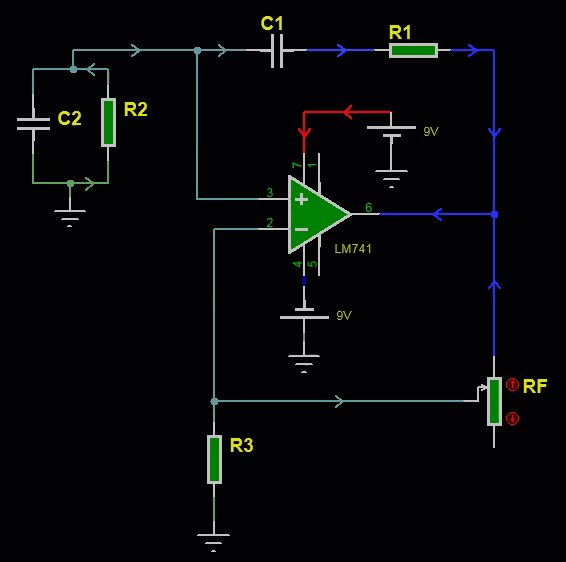# LM741 Wien bridge oscillator design

The LM741 operational amplifier is a widely used component in electronic circuit design. One of the applications of the LM741 op-amp is in the design of Wien bridge oscillators. Here, we will show how to design of a Wien bridge oscillator using the LM741 op-amp and discuss its applications.

### Wien Bridge Oscillator

A Wien bridge oscillator is a type of oscillator that generates a sinusoidal output waveform at a specific frequency. It is capable of generating perfectly sinusoidal waveform within frequency range from 20KHz to 100KHz. The Wien bridge oscillator uses feedback network that uses a combination of resistors and capacitors but the RC feedback network does not produce any phase shift unlike the LM741 Phase Shift Oscillator. The feedback network is connected to non-inverting terminal of the operational amplifier. The The LM741 op-amp is used to provide the necessary gain to sustain oscillation. The required gain for Wien Bridge oscillator is equal to greater than 2.

Here is the schematic diagram of the LM741 Wien bridge oscillator:As can be see from the above circuit diagram, there are two RC network made up of R1, C1 which are in series and another RC network made up of R2, C2 which are connected in parallel. The resistors RF and R3 sets the gain of the LM741 non-inverting amplifier. The circuit is powered using dual power supply but the circuit can also be set up to use LM741 with single power supply.

The frequency of the Wein Bridge oscillator is given by,

$$f= \frac{1}{2 \pi \sqrt{R_1R_2C_1C_2}}$$   -------->(1)

Usually, in practical design, equal resistors(R=R1=R2) and equal capacitors(C=C1=C2) are used and in this case the frequency equation(1) reduces to,

$$f= \frac{1}{2 \pi RC}$$   -------->(2)

With equal resistors and capacitors, it can be deduced that for Wein bridge oscillator the feedback fraction$$\beta$$ is equal to 1/3, that is,

$$\beta = \frac{1}{3}$$    ------->(3)

The positive sign of $$\beta$$ indicates that the phase shift by the feedback network is 0 degree,

Similarly the gain required by Wein Bridge oscillator can be obtained using Barkhausen criteria.

$$A \beta \ge 1$$      -------->(4)

Using (3) we have,

$$A \ge 3$$      -------->(5)

Since the Wein bridge oscillator is connected in non-inverting amplifier configuration we have,

$$A = 1+ \frac{R_F}{R_3}$$      -------->(6)

Combining (5) and (6) we get,

$$1+ \frac{R_F}{R_3} \ge 3$$

$$\frac{R_F}{R_3} \ge 2$$

or, $$R_F \ge 2R_3$$   -------->(7)

These component values can be computed directly using the online Wein Bridge oscillator calculator.

### Steps to design a Wien bridge oscillator

Here are the steps to design a Wien bridge oscillator using the LM741 op-amp:

• Choose oscillation frequency

Let the frequency of oscillation be 50KHz

• Choose the values of R and C

Assuming we are using equal value resistors and capacitors we need to choose either a values for R or C to produce the desired frequency of oscillation. Let's say we use 1nF capacitor then we can use the Wein Bridge oscillator frequency formula in equation (1) and solve for resistor R.

$$R = \frac{1}{2 \pi f C}$$    -------->(8)

Subtituting values we get,

$$R = \frac{1}{2 \pi \times 50KHz \times 1nF } = 3.18 KΩ$$

• Calculate the feedback resistor

We can calculate the feedback resistor value by using equation(7) by selecting value for R3. Let us use R3=1KΩ.

$$R_F \ge 2R_3 \ge 2 \times 1KΩ \ge 2KΩ$$

#### Construct the circuit:

The circuit can be constructed by connecting the feedback network to the non-inverting input of the op-amp and connecting the output of the op-amp to the input of the feedback network. The circuit is powered using a dual power supply. The values for the components were already calculated above. The completed circuit diagram of op-amp oscillator with LM741 is shown below.

### Application of LM741 Wien bridge oscillator

The LM741 Wien bridge oscillator has a wide range of applications, including:

• Audio applications: The LM741 Wien bridge oscillator is commonly used in audio applications, such as tone generators, filters, and equalizers.
• Testing and measurement: The LM741 Wien bridge oscillator is used in testing and measurement applications to generate precise and stable sine waves.
• Communication systems: The LM741 Wien bridge oscillator is used in communication systems to generate carrier signals for modulation.

Video demonstration

The following video shows how the LM741 based Wien Bridge oscillator circuit works.

In conclusion, the LM741 op-amp can be used to design a Wien bridge oscillator that generates a sinusoidal output waveform at a specific frequency. By choosing the appropriate values of R and C and the required gain, the frequency of oscillation can be achieved. The LM741 Wien bridge oscillator is a versatile and reliable design that has a wide range of applications in electronic circuit design.